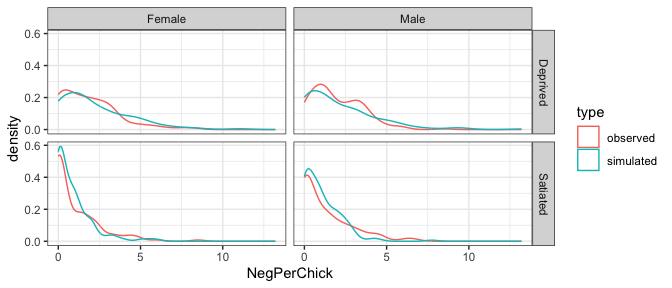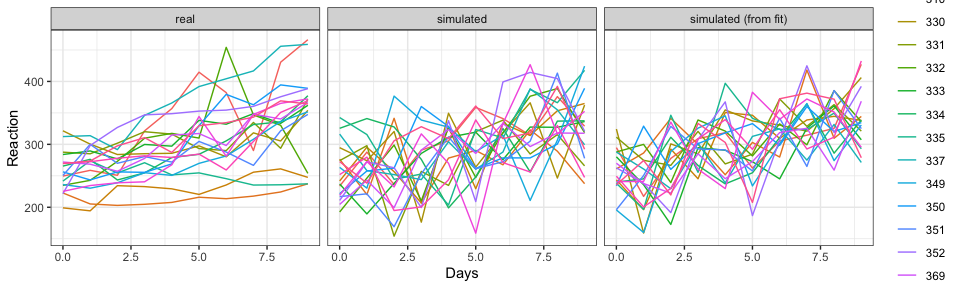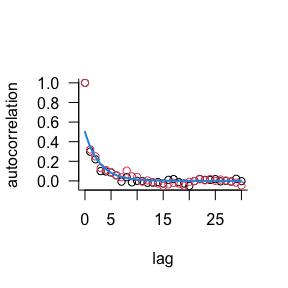# Simulate from a fitted glmmTMB model or a formula

## Simulating from a fitted model

glmmTMB has the capability to simulate from a fitted model. These simulations resample random effects from their estimated distribution. In future versions of glmmTMB, it may be possible to condition on estimated random effects.

library(glmmTMB)
library(ggplot2); theme_set(theme_bw())

Fit a typical model:

data(Owls)
owls_nb1 <- glmmTMB(SiblingNegotiation ~ FoodTreatment*SexParent +
(1|Nest)+offset(log(BroodSize)),
family = nbinom1,
ziformula = ~1, data=Owls)

Then we can simulate from the fitted model with the simulate.glmmTMB function. It produces a list of simulated observation vectors, each of which is the same size as the original vector of observations. The default is to only simulate one vector (nsim=1) but we still return a list for consistency.

simo=simulate(owls_nb1, seed=1)
Simdat=Owls
Simdat$SiblingNegotiation=simo[] Simdat=transform(Simdat, NegPerChick = SiblingNegotiation/BroodSize, type="simulated") Owls$type = "observed"
Dat=rbind(Owls, Simdat) 

Then we can plot the simulated data against the observed data to check if they are similar.

ggplot(Dat,  aes(NegPerChick, colour=type))+geom_density()+facet_grid(FoodTreatment~SexParent)## Simulating from scratch (de novo)

what if you want to simulate data with specified parameters in the absence of a data set, for example for a power analysis?

glmmTMB has a simulate_new function that can handle this case; the hardest part is understanding the meaning of the parameter values, especially for random-effects covariances.

### example 1: linear regression

For the first example we'll simulate something that looks like the classic "sleep study" data, using the sleepstudy data set for structure and covariates. The conditional-fixed effects parameters (beta) are standard regression parameters (intercept and slope): we use 250 and 10, which are close to the values from the actual data. The only other parameter, betad, is the log of the dispersion parameter, which in the specific case of the Gaussian (default) family is the log of the conditional (residual) variance; the standard deviation from a simple regression on these data1 is approximately 50, so we use 2*log(50).

data("sleepstudy", package = "lme4")
set.seed(101)
ss_sim <- transform(sleepstudy,
Reaction = simulate_new(~ Days,
newdata = sleepstudy,
family = gaussian,
newparams = list(beta = c(250, 10),
betad = 2*log(50)))[])

For comparison, we'll also fit the model and use the built-in simulation method:

ss_fit <- glmmTMB(Reaction ~ Days, sleepstudy)
ss_simlm <- transform(sleepstudy,
Reaction = simulate(ss_fit)[])

Comparing against the real data set:

library(ggplot2); theme_set(theme_bw())
ss_comb <- rbind(data.frame(sleepstudy, sample = "real"),
data.frame(ss_sim, sample = "simulated"),
data.frame(ss_simlm, sample = "simulated (from fit)")
)
ggplot(ss_comb, aes(x = Days, y = Reaction, colour = Subject)) +
geom_line() +
facet_wrap(~sample)The simulated data have about the right variability, but in contrast to the real data have no among-subject variation.

### example 2: random effects (including correlations)

The next example will be more complex, getting into the nuts and bolts of how to translate random effects covariances into the terms that glmmTMB expects.

The hardest piece is probably translating correlation parameters. The "covariance structures" vignette has more details on how correlation matrices are parameterized, and the put_cor() function is a general translator, but for the specific case of 2x2 correlation matrices (i.e. with a single correlation parameter), a correlation $$\rho$$ corresponds to a glmmTMB parameter of $$\rho/\sqrt{1-\rho^2}$$. Here's a utility function:

rho_to_theta <- function(rho) rho/sqrt(1-rho^2)
## tests
stopifnot(all.equal(get_cor(rho_to_theta(-0.2)), -0.2))
stopifnot(all.equal(rho_to_theta(-0.2), put_cor(matrix(c(1,-0.2,-0.2,1), 2))))

Setting up metadata/covariates (tools in the faux package may also be useful for this):

n_id <- 10
dd <- expand.grid(trt = factor(c("A", "B")),
id = factor(1:n_id),
time = 1:6)

We'll set up some reasonable fixed effects. When in doubt about the order of fixed effect parameters, use model.matrix() to check:

form1 <- ~trt*time+(1+time|id)
colnames(model.matrix(lme4::nobars(form1), data = dd))
##  "(Intercept)" "trtB"        "time"        "trtB:time"
## intercept, trtB effect, slope, trt x slope interaction
beta_vec <- c(1, 2, 0.1, 0.2)

We'll set SDs such that the average coeff var = 1 (SD = mean for among-subject variation in intercept and slope). As described in the "covstruct" vignette, the parameter vector for a random-effect covariance consists of the log-standard-deviations followed by the scaled correlations. Finally we'll set the dispersion parameter for the negative binomial conditional distribution to 1 (more detail on the betad parameterization for different families is given in ?sigma.glmmTMB).

sdvec <-  c(1.5,0.15)
corval <- -0.2
thetavec <- c(log(sdvec), rho_to_theta(corval))
par1 <- list(beta = beta_vec,
theta = thetavec)

Now simulate:

dd$y <- simulate_new(form1, newdata = dd, seed = 101, family = nbinom2, newparams = par1)[] range(dd$y)
##    0 598

For comparison, we'll do this by hand (with some help from lme4 machinery). lme4 parameterizes covariance matrices by the lower triangle of the Cholesky factor rather than using glmmTMB's method ...

library(lme4)
## Loading required package: Matrix
set.seed(101)
X <- model.matrix(nobars(form1), data  = dd)
## generate random effects values
rt <- mkReTrms(findbars(form1),
model.frame(subbars(form1), data = dd))
Z <- t(rt$Zt) ## construct covariance matrix Sigma <- diag(sdvec) %*% matrix(c(1, corval, corval, 1), 2) %*% diag(sdvec) lmer_thetavec <- t(chol(Sigma))[c(1,2,4)] ## plug values into Cholesky factor of random effects covariance matrix rt$Lambdat@x <- lmer_thetavec[rt$Lind] u <- rnorm(nrow(rt$Lambdat))
b <- t(rt$Lambdat) %*% u eta <- drop(X %*% par1$beta + t(rt$Zt) %*% b) mu <- exp(eta) y <- rnbinom(nrow(dd), size = 1, mu = mu) range(y) ## range varies a lot ##  0 1484 Alternatively we could have generated the random effects with: b <- MASS::mvrnorm(1, mu = rep(0,2*n_id), Sigma = Matrix::.bdiag(replicate(n_id, Sigma, simplify = FALSE))) ### example 3: AR1 model We will simulate a single time series with AR1 structure, with a nugget (measurement error) variance $$\sigma^2_n = 1.0$$, an autoregressive variance of $$\sigma^2_a = 1$$, and an autoregressive parameter of $$\phi = 0.7$$, First by brute force, using the code from the "covariance structures" vignette: set.seed(101) n <- 1000 ## Number of time points x <- MASS::mvrnorm(mu = rep(0,n), Sigma = .7 ^ as.matrix(dist(1:n)) ) ## Simulate the process using the MASS package ## as.matrix(dist(1:n)) constructs a banded matrix with m_{ij} = abs(i-j) y <- x + rnorm(n) ## Add measurement noise/nugget dat0 <- data.frame(y, times = factor(1:n, levels=1:n), group = factor(rep(1, n))) Now using simulate_new() with matching parameters beta = 0 (the only fixed effect is the intercept, which we set to zero); betad = 0 (the log-variance of the measurement error [note Gaussian family uses log-variance rather than log-SD parameterization, although in this case it doesn't make any difference ...]); theta = 0 (log-SD of autoregressive variance); and theta specifying a correlation parameter $$\phi = 0.7$$ (see "Covariance structures" vignette for details). phi_to_AR1 <- function(phi) phi/sqrt(1-phi^2) s2 <- simulate_new(~ ar1(times + 0 | group), newdata = dat0, seed = 101, newparams = list( beta = 0, betad = 0, theta = c(0, phi_to_AR1(0.7))) )[] With a nugget variance of $$\sigma^2_n = 1.0$$, an autoregressive variance of $$\sigma^2_a = 1$$, and an autoregressive parameter of $$\phi = 0.7$$, we expect the ACF to be $$\sigma^2_a/(\sigma^2_a + \sigma^2_n) \phi^d$$ . a1 <- drop(acf(dat0$y, plot = FALSE)$acf) a2 <- drop(acf(s2, plot = FALSE)$acf)
par(las = 1, bty = "l")
matplot(0:(length(a1)-1), cbind(a1, a2), pch = 1,
ylab = "autocorrelation", xlab = "lag")
curve(0.7^x/2, add = TRUE, col = 4, lwd = 2)The precise curves are different (because the multivariate normal deviates are generated in a different way), but the ACFs match.

## FIXME/TO DO

• more examples! especially more complex/unavailable in lme4 (spatial, ZI, etc.). If necessary, more details on parameterizations (shape/scale for spatial cov structures, etc.)

1. I realize this violates the assumption of de novo simulation that we don't know what the real data looks like yet ...